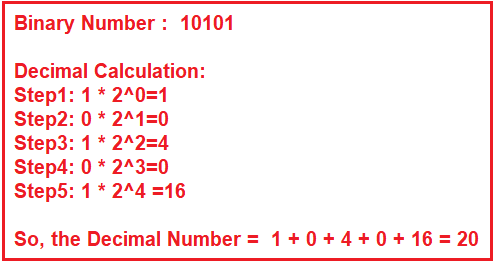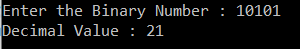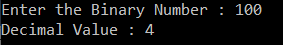# Binary to Decimal Conversion in C#

## Binary to Decimal Conversion in C# with Examples

In this article, I am going to discuss the Binary to Decimal Conversion in C# with Examples. Please read our previous article where we discussed the Decimal to Binary Conversion Program in C# with examples. In C#, we can easily convert a binary number (base-2 (i.e. 0 or 1)) into decimal number (base-10 (i.e. 0 to 9)).

##### How to Convert Binary Number to Decimal Number in C#?

The following diagram shows how to convert a binary number i.e. 10101 into its equivalent decimal number i.e. 21.##### Implementing Binary to Decimal Conversion Program in C#:

In the following example, first, we extract the digits of a given binary number starting from the rightmost digits using the modulus operator. Once we extract the number, then we multiply the digit with the proper base i.e. power of 2 and add the result into the decimal value variable. In the end, the decimal value variable will hole the required decimal number.

```using System;
namespace LINQDemo
{
class Program
{
static void Main(string[] args)
{
Console.Write("Enter the Binary Number : ");
int decimalValue = 0;

// initializing base1 value to 1, i.e 2^0
int base1 = 1;

while (binaryNumber > 0)
{
int reminder = binaryNumber % 10;
binaryNumber = binaryNumber / 10;
decimalValue += reminder * base1;
base1 = base1 * 2;
}
Console.Write(\$"Decimal Value : {decimalValue} ");

}
}
}
```
###### Output:Please Note: The above program works with binary numbers within the range of integers. If you want to work with long binary numbers such as 20 bits or 30 bit, then you need to use the string variable to store the binary numbers.

##### Binary to Decimal Conversion using Convert.ToInt32() method:

In the following example, we are using the ToInt32 method to convert a binary number to a decimal number. This excepts two parameters. The first parameter is the string representation of the binary number and the second parameter is the base value i.e. in our case it is 2.

```using System;
namespace LINQDemo
{
class Program
{
static void Main(string[] args)
{
Console.Write("Enter the Binary Number : ");

int decimalValue = Convert.ToInt32(binaryNumber.ToString(), 2);
Console.Write(\$"Decimal Value : {decimalValue} ");